Refer to our Texas Go Math Grade 1 Answer Key Pdf to score good marks in the exams. Test yourself by practicing the problems from Texas Go Math Grade 1 Module 9 Assessment Answer Key.

Vocabulary

Question 1.
circle the penny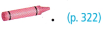Circled the penny with pink

Question 2.
circle the nickelCircled the Nickel with yellow

Question 3.
circle the quarter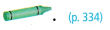Circled the quarter with green

Question 4.
1. circle the dimeCircled the dime with blueExplanation:
Identification of coins.

Concepts and Skills

Question 5.
Write the value of each coin. TEKS 1.4.B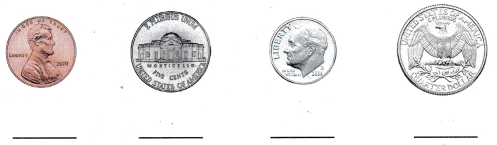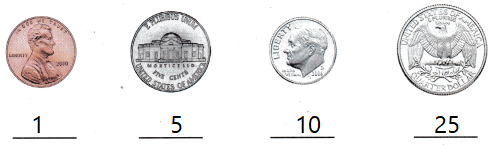Explanation:
Written the value of each coin in cents

Question 6.
Describe how a dime and a penny are related. TEKS 1.4.A
1 dime = 10 cents
10 cents = 10 pennies
Explanation:
They can be related with cents.

Question 7.
Describe how a quarter and a nickel are related. TEKS 1.4.A
1 quarter = 25
1 nickel = 5
Explanation:
1 quarter = 5 nickels

Texas test Prep

Question 8.
What is the total value? TEKS 1.4.A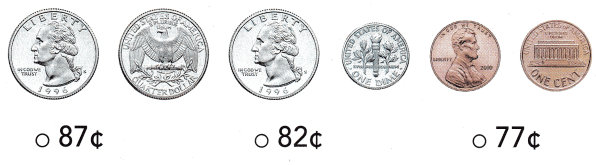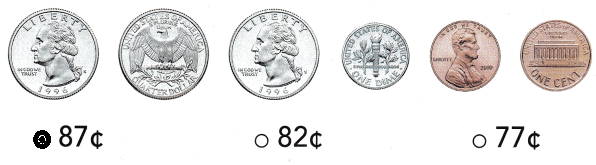Explanation:
3 x 25 = 75
1 x 10 = 10
2 x 1 = 2
75 + 10 + 2 = 87

Question 9.
Mandy has 8 nickels. Which shows the same amount in a different way? TEKS 1.4.C